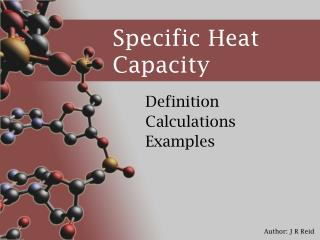DownloadDownload PresentationSpecific Heat Capacity

# Specific Heat Capacity

Télécharger la présentation## Specific Heat Capacity

- - - - - - - - - - - - - - - - - - - - - - - - - - - E N D - - - - - - - - - - - - - - - - - - - - - - - - - - -
##### Presentation Transcript

1. Specific Heat Capacity Definition Calculations Examples

2. Definition • Specific heat capacity is also known as ‘specific heat’ • It is the energy required to raise 1 gram of a chemical by 1 degree Celsius • It has a unit of J°C-1g-1 (or Joules per degree Celsius per gram) • Some substances need a lot of energy to raise their temperature, others need less: • Water = 4.18 • Ethanol = 2.44 • Lead = 0.13

3. Other Examples • Aluminium = 0.90 • Water       = 4.18 • Carbon       = 0.72 • ethanol = 2.44 • Copper       = 0.39 • Sulphuric acid = 1.42 • Lead       = 0.13 • Sodium chloride = 0.85 • Mercury       = 0.14

4. Calculations – Example 1 • What amount of heat energy is required to raise 20g of water by 30°C? • First we find the heat capacity for water • 4.18 J°C-1g-1 • Next we multiply by the mass (in grams) • 4.18 x 20g = 83.6 J°C-1 (Joules per degree) • Next we multiply by the temperature change • 83.6 x 30°C = 2508J (or 2.508kJ)

5. Calculation – Example 2 • What amount of heat energy is required to boil 30 millilitres of water from a temperature of 25°C • What mass of water will be used? (Note: 1mL of water = 1g • What temperature change will be needed? Note: Volume (litres) and amount (moles) need to be converted into grams before being used in this type of calculation

6. Exam Practice - 2006 Can’t see the exam paper below? Go to the NCEA website and search for 90780 • Have a go at Questions:

7. Exam Practice - 2007 Can’t see the exam paper below? Go to the NCEA website and search for 90780 • Have a go at Questions: